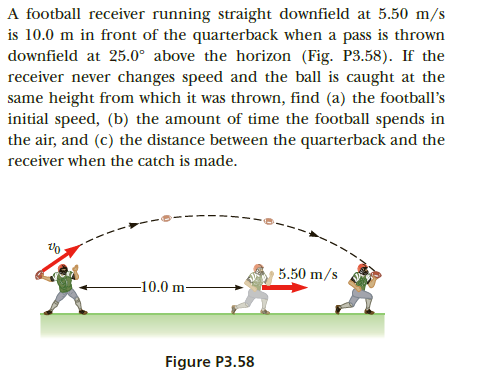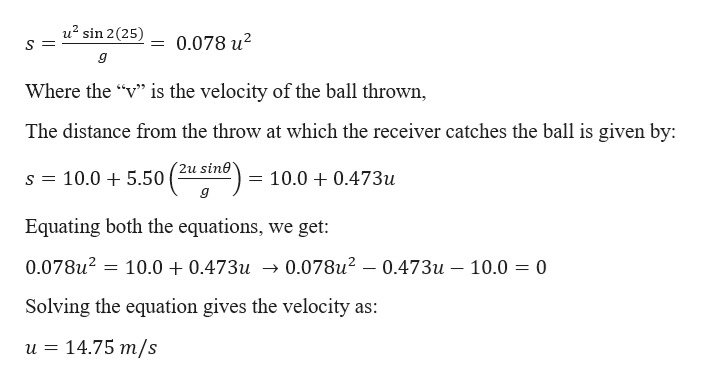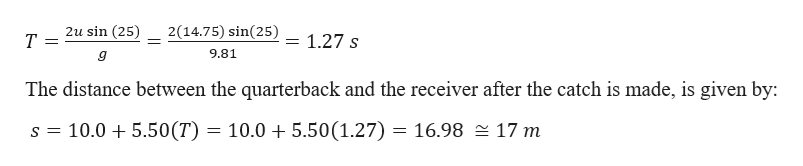# A football receiver running straight downfield at 5.50 m/sis 10.0 m in front of the quarterback when a pass is throwndownfield at 25.0° above the horizon (Fig. P3.58). If thereceiver never changes speed and the ball is caught at thesame height from which it was thrown, find (a) the football'sinitial speed, (b) the amount of time the football spends inthe air, and (c) the distance between the quarterback and thereceiver when the catch is made.5.50 m/s-10.0 m-Figure P3.58

Question
1 viewshelp_outlineImage TranscriptioncloseA football receiver running straight downfield at 5.50 m/s is 10.0 m in front of the quarterback when a pass is thrown downfield at 25.0° above the horizon (Fig. P3.58). If the receiver never changes speed and the ball is caught at the same height from which it was thrown, find (a) the football's initial speed, (b) the amount of time the football spends in the air, and (c) the distance between the quarterback and the receiver when the catch is made. 5.50 m/s -10.0 m- Figure P3.58 fullscreen
check_circle

Step 1

Given information:

Speed of the receiver (vr) = 5.50 m/s

Angle at which the ball is thrown (θ) = 250

The distance of the receiver from the throw (d) = 10.00 m

Step 2

Let “T” be the time spent by the ball in air, then the horizontal distance travelled by the ball in this time is given by:help_outlineImage Transcriptioncloseu? sin 2(25) = 0.078 u? Where the "" is the velocity of the ball thrown, The distance from the throw at which the receiver catches the ball is given by: 2u sine 10.0 + 5.50 ( :10.0 + 0.473u Equating both the equations, we get: 0.078u? = 10.0 + 0.473u → 0.078u? – 0.473u – 10.0 = 0 Solving the equation gives the velocity as: u = 14.75 m/s fullscreen
Step 3

The total time of flight is g...help_outlineImage Transcriptionclose2u sin (25) т 2(14.75) sin(25) 1.27 s 9.81 The distance between the quarterback and the receiver after the catch is made, is given by: s = 10.0 + 5.50(T) = 10.0 + 5.50(1.27) = 16.98 = 17 m fullscreen

### Want to see the full answer?

See Solution

#### Want to see this answer and more?

Solutions are written by subject experts who are available 24/7. Questions are typically answered within 1 hour.*

See Solution
*Response times may vary by subject and question.
Tagged in

### Kinematics